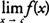## Intuitive Definition

The concept of the limit of a function is essential to the study of calculus. It is used in defining some of the most important concepts in calculus—continuity, the derivative of a function, and the definite integral of a function.

The limit of a function f( x) describes the behavior of the function close to a particular x value. It does not necessarily give the value of the function at x. You write, which means that as x “approaches” c, the function f( x) “approaches” the real number L (see Figure 1).Figure 1 The limit of f(x) as x approaches c.

In other words, as the independent variable x gets closer and closer to c, the function value f( x) gets closer to L. Note that this does not imply that f( c) = L; in fact, the function may not even exist at c (Figure 2) or may equal some value different than L at c (Figure 3).Figure 2 f ( c) does not exist, butdoes.

If the function does not approach a real number L as x approaches c, the limit does not exist; therefore, you writeDNE (Does Not Exist). Many different situations could occur in determining that the limit of a function does not exist as x approaches some value.

Back to Top
A18ACD436D5A3997E3DA2573E3FD792A